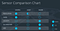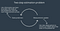# UDACITY SDCE Nanodegree Term 2: Kalman Filters for Sensor Fusion

Fusing together measurements from heterogeneous sensors found in an autonomous vehicle enables more accurate tracking of the objects in the environment. In the first module of Term 2 of the SDCE Nanodegree we were tasked with implementing both the extended Kalman Filter as well as the unscented Kalman Filter in C++ to track the position of a single object.

# Sensors

Among the most popular sensors used in autonomous vehicles we have lidars, radars, and cameras. Each sensor has its own strength and weaknesses. Thus it is important to develop sensor fusion approaches in order to take advantage of each the sensors.Sensor Comparison Chart (Source: UDACITY)

# Kalman Filters

The traditional Kalman Filter is an algorithm that is used to produce estimates for unknown variables given a series of measurements. It uses Bayesian inference to estimate a joint probability distribution over the variables for a considered period. Essentially, the Kalman Filter maintains a mean vector and co-variance matrix for the estimated variables that represent the belief for each possible state through Gaussian functions. The algorithm and its variants (Extended and Unscented Kalman Filters) iterate on two main cycles: Measurement Update and State Prediction. In the measurement update step we use new observations from the sensors to update our belief estimation for the unkown variables. Then in the state prediction step we predict using a system model (e.g., motion model) the future value of the unknown variables.Basic Kalman FIlter Steps (Source: UDACITY)

In the case where multiple sensors are used then each sensor has its own measurement update scheme. The belief about the state of the unkown variables is updated asynchronously each time the measurement is received regardless of the sensor sources.

# Extended Kalman Filters (EKF)

While the traditional Kalman Filter has many applications its main drawback is that it assumes a linear measurement model and state prediction model. This may be a fair assumption under specific conditions but in general real-word systems are non-linear. For example. the lidar sensor has a linear measurement update model, whereas, the radar sensor has a non-linear update model. The Extended Kalman Filter attempts to solve such problems by linearizing the non-linear state transition functions using Taylor Expansion around the mean location of the original function. In addition, the linearization requires that the Jacobian of the state transition is computed.

# Unscented Kalman Filters (UKF)

While Estended Kalman Filters work well for linear models, the real world rarely behaves in a linear fashion. ENTER the Unscented Kalman Filters! With these filters we are able to accurately model non-linear phenomena. Instead of linearizing a non-linear function UKF uses the unscented transformation to approximate the belief probability distribution. In this way it performs better than linearization and does not require to compute Jacobians.

The main jist of UKF is to find the multivariate Gaussian distribution that approximated the real belief distribution. The predicted states may not be normaly distributed but the UKF assumes that they are. Specifically, the Gaussian distribution approximated the real distribution as close as possible with respect to its mean and covariance matrix. The pipeline for UKF is to first generate some samples sigma points that define the Gaussian. Then to predict new sigma points based on the system model (e.g., motion model). Using the new sigma points we then predict the mean and covariance of the Gaussina. These three steps constitute the Prediction Step for the UKF. The Measurements Update follows next, which includes Predicting the new measurements and updating the state belief.

# Implementation in C++

The goals / steps of this project were the following:
• Use simulated lidar and radar measurements to track the position and velocity of an object.
• Optionally, use a simulator to visualize measurements and tracked object positions.

We worked with a kalman filter class and had to implement the prediction and update steps for the two versions of the kalman filter and receiving data from both Lidar and Radar sensors. It is important to note here that the lidar measurement model is linear hence, we can utilize the standard kalman filter model for this sensor. The developed algorithmic pipeline in C++ is capable of tracking vehicles and estimating their position within acceptable root mean square error. Below is a video of the extended kalman filter that tracks a vehicle and estimates its velocity and position.

# Summary

A standard Kalman filter can only handle linear equations. Both the extended Kalman filter and the unscented Kalman filter allow you to use non-linear equations; the difference between EKF and UKF is how they handle non-linear equations. But the basics are the same: initialize, predict, update.

PhD in Computer Engineering, Self-Driving Car Engineering Nanodegree, Computer Vision, Visual Perception and Computing

## More from Christos Kyrkou

PhD in Computer Engineering, Self-Driving Car Engineering Nanodegree, Computer Vision, Visual Perception and Computing